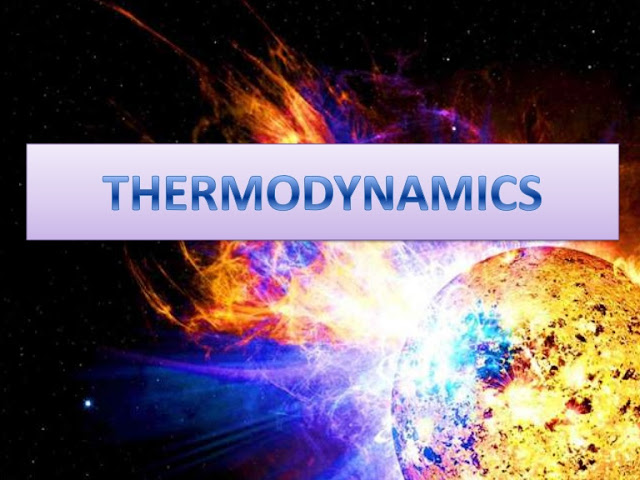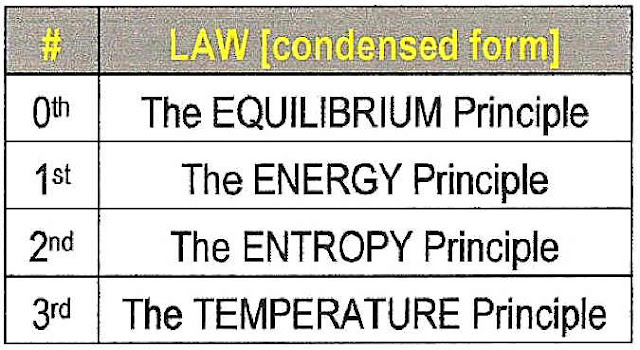## Translate

### Thermodynamics| Laws |System | Equations

#### Thermodynamics:#### Father Of Thermodynamics:

The applications of the laws of thermodynamics has led to the discovery of important machines like automobile, ships, airplanes , refrigerator, air-conditioner, etc. A young man, Nicholas Leonard Sadi Carnot of 36 years of age is considered to be the father of thermodynamics.

#### DEFINITION:

Thermodynamics is the science of relationship between Energy and Material System.
OR
Thermodynamic is the science of the regularities governing process of Energy Converstion.

#### Thermodynamic System:

System: A system is a finite quantity of matter or a prescribed region of space.

The Thermodynamics system may be classified into three groups:

1. Closed System.
2. open System.
3. Isolated system.

#### Following are the four laws of thermodynamics :#### 1. Zeroth law of thermodynamics :

The Zeroth law states that if two bodies are in thermal equilibrium with a third body, they are also in thermal equilibrium with each other.
Thermal equilibrium means that when two bodies are brought into contact with each other and separated by a barrier that is permeable to heat, there will be no transfer of heat from one to the other.

#### 2. First law of thermodynamics :

The first law throws light on concept of internal energy.This law states that the heat and mechanical work are mutually convertible. According to this law, a defined amount of mechanical work is needed to produce a definite amount of heat and vice versa. The first law of thermodynamics is an expression of the principle of conservation of energy.This law also states that the energy can neither be created nor destroyed, through it can be transformed from one form to another. According to this law, the energy due to heat supplied(Q),Must be balanced by the external work done(W) plus the gain internal energy(E)due to rise temperature.

In other words,

Q=W+E

#### 3. Second law of thermodynamics :

Heat cannot be sudden flow from a colder location to a hotter location. This law states that the limit of converting heat into work and introduces the principle of increasing of Entropy.

According to Clausius, this law may be stated as “It is impossible for self-acting machine working in a cyclic process, to transfer heat from a body at a lower temperature to a body at a higher temperature without the aid of an external agency”.

The second law of thermodynamics has also been stated by Kenvin-planck as “It is impossible to construct an engine working on a cyclic process, whose sole purpose is to convert heat energy into work.” According to this statement, the second law of thermodynamics is sometimes called as “law of degradation of energy”.
There are many versions of the second law, but they all have the same effect, which is to explain the phenomenon of irreversibility in nature.

#### 4. Third law of thermodynamics:

The Third law defines the absolute zero of entropy.

The third law of thermodynamics is a statistical law of nature regarding entropy and the impossibility of reaching absolute zero of temperature.

Absolute zero, at which all activity would stop if it were possible to happen, is −273.15 °C (degrees Celsius), or −459.67 °F (degrees Fahrenheit) or 0 K (kelvin).

These laws are based on the experimental observation and have no mathematical proof. Like all physical laws, these laws are based on Logical Reasoning.